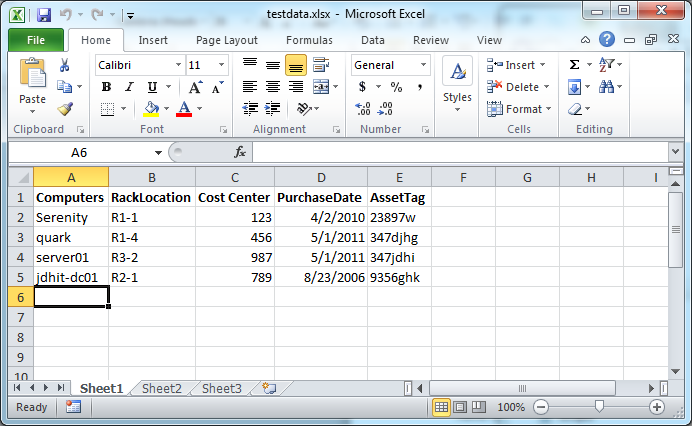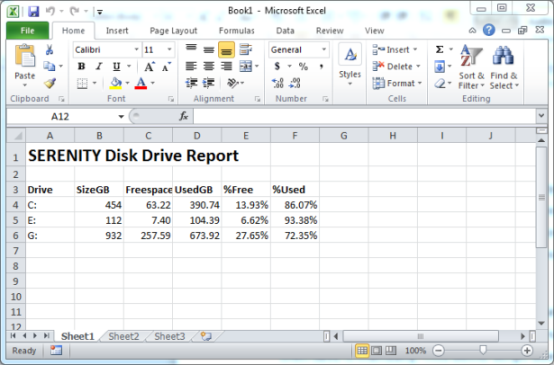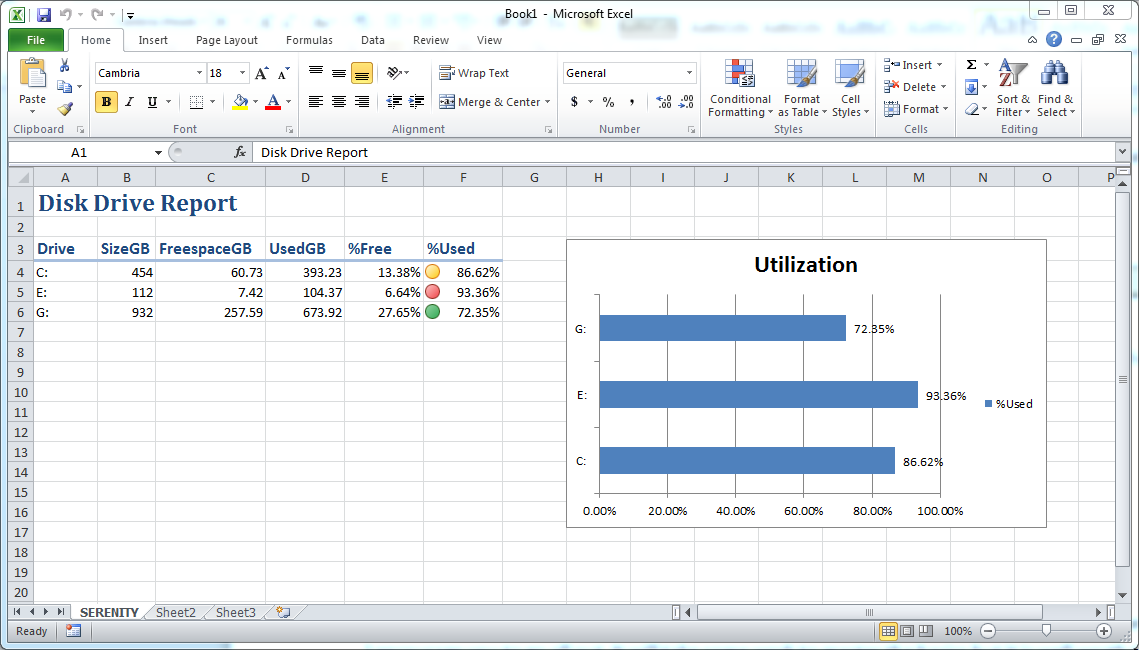# Excel 列号和数字互相转换

Excel 的列号是采用“A”、“B”……“Z”、“AA”、“AB”……的方式编号。但是我们在自动化操作中，往往希望用数字作为列号。我们可以用 PowerShell 来实现 Excel 的列号和数字之间的互相转换。

## 需求归纳

Excel 列号 -> 数字

``````A   1
AB  28
AC  29
``````

``````1   A
2   B
24  Y
26  Z
27  AA
28  AB
``````

# 算法分析

• Excel 列号 -> 数字
• 用 ASCII 编码对输入的字符串解码，得到一个数字型数组。
• 用 26 进制对数组进行处理（逐位 *= 26，然后累加）。
• 数字 -> Excel 列号
• 用 26 进制对数字进行处理（不断地 /= 26，取余数），得到数字型数组。
• 将数字型数组顺序颠倒。
• 用 ASCII 编码对数字型数组编码，得到 Excel 风格的列号。

# 源代码

``````function ConvertFrom-ExcelColumn (\$column) {
\$result = 0
\$ids = [System.Text.Encoding]::ASCII.GetBytes(\$column) | foreach {
\$result = \$result * 26 + \$_ - 64
}
return \$result
}

function ConvertTo-ExcelColumn (\$number) {
\$ids = while (\$number -gt 0) {
(\$number - 1) % 26 + 1 + 64
\$number = [math]::Truncate((\$number - 1) / 26)
}

[array]::Reverse(\$ids)
return [System.Text.Encoding]::ASCII.GetString([array]\$ids)
}
``````

``````echo "A`t\$(ConvertFrom-ExcelColumn A)"
echo "AB`t\$(ConvertFrom-ExcelColumn AB)"
echo "AC`t\$(ConvertFrom-ExcelColumn AC)"

echo ''

@(1..2) + @(25..28) | foreach {
echo "\$_`t\$(ConvertTo-ExcelColumn \$_)"
}
``````

``````A   1
AB  28
AC  29

1   A
2   B
25  Y
26  Z
27  AA
28  AB
``````

# 在PowerShell中操作Word - 使用格式化样式

``````PS C:\>\$word=new-object -ComObject "Word.Application"
PS C:\> \$selection=\$word.Selection
``````

``````PS C:\> \$selection.Font.Color="wdColorGreen"
PS C:\> \$selection.TypeText((Get-Date))
``````

``````PS C:\> [enum]::GetNames([microsoft.office.interop.word.wdcolor])
``````

``````PS C:\ >\$selection.font.Color="wdColorAutomatic"
PS C:\> \$selection.TypeParagraph()
``````

``````\$selection.Font.Size=12
\$selection.TypeText("Operating System Information for \$(\$os.CSName)")
``````

``````PS C:\> \$selection.Font.Size=10
PS C:\> \$selection.Font.Name="Consolas"
PS C:\> \$selection.TypeText((\$os | Select -Property \$props | Out-String))
``````

``````PS C:\> \$selection.Font.size=8
PS C:\> \$selection.Font.Name="Calibri"
PS C:\> \$selection.Font.Italic=\$True
PS C:\> \$by="Report created by \$env:userdomain\\$env:username"
PS C:\> \$selection.TypeText(\$by)
``````

``````\$selection.Style="Title"
\$selection.TypeText("Operating System Report")
\$selection.TypeParagraph()
``````

``````\$doc.Styles | select NameLocal
``````

# 创建Word应用程序对象

PowerShell可以通过COM接口控制Word应用程序。有趣的地方是，虽然您可以交互式地做所有的操作，但我希望您最终能够用脚本操作一切。我们从创建一个Word程序对象开始。

``````PS C:\> \$word=new-object -ComObject "Word.Application"
``````

``````PS C:\> \$doc=\$word.documents.Add()
``````

``````PS C:\> \$word.Visible=\$True
``````

``````PS C:\> \$selection=\$word.Selection
``````

# 用PowerShell在文档中插入文本

``````PS C:\> \$selection.TypeText((Get-Date))
``````

``````PS C:\> \$selection.TypeParagraph()
``````

``````PS C:\> \$os=Get-WmiObject -class win32_OperatingSystem
PS C:\> \$selection.TypeText("Operating System Information for \$(\$os.CSName)")
``````

``````PS C:\> \$os.properties | select Name | foreach -begin {\$props=@()} -proc {\$props+="\$(\$_.name)"}
``````

``````PS C:\> \$selection.TypeText((\$os | Select -Property \$props | Out-String))
``````

``````PS C:\> \$doc.SaveAs([ref]"c:\work\osreport.docx")
PS C:\> \$doc.Close()
``````

``````PS C:\> \$word.quit()
``````

# 读取数据

``````\$xl=New-Object -ComObject "Excel.Application"
````````````\$wb=\$xl.Workbooks.Open(\$file)
\$ws=\$wb.ActiveSheet
``````

\$ws对象是我们对数据最重要的的引用点。我需要用的数据从A2单元格开始。在我的测试环境中，我也许知道我需要处理多少行，但是既然我知道从哪儿开始，我可以用一个Do循环来读取每一行，获取数据，进行进一步操作。

``````\$Row=2

do {
\$data=\$ws.Range("A\$Row").Text
...
``````

``````if (\$data) {
Write-Verbose "Querying \$data"
\$ping=Test-Connection -ComputerName \$data -Quiet
``````

``````if (\$Ping) {
\$OS=(Get-WmiObject -Class Win32_OperatingSystem -Property Caption -computer \$data).Caption
}
else {
\$OS=\$Null
``````

``````New-Object -TypeName PSObject -Property @{
Computername=\$Data.ToUpper()
OS=\$OS
Ping=\$Ping
Location=\$ws.Range("B\$Row").Text
AssetAge=((Get-Date)-(\$ws.Range("D\$Row").Text -as [datetime])).TotalDays -as [int]
}
``````

loop循环的最后一步是使行计数器自增1。

``````\$Row++
} While (\$data)
``````

``````\$xl.displayAlerts=\$False
\$wb.Close()
\$xl.Application.Quit()
``````

``````PS C:\scripts> .\Demo-ReadExcel.ps1

AssetAge     : 687
Ping         : True
Computername : SERENITY
Location     : R1-1
OS           : Microsoft Windows 7 Ultimate

AssetAge     : 293
Ping         : True
Computername : QUARK
Location     : R1-4
OS           : Microsoft Windows 7 Professional

AssetAge     : 293
Ping         : False
Computername : SERVER01
Location     : R3-2
OS           :

AssetAge     : 2005
Ping         : True
Computername : JDHIT-DC01
Location     : R2-1
OS           : Microsoft(R) Windows(R) Server 2003, Enterprise Edition
``````

# 创建一个简单的报表

Microsoft Excel包含一个非常复杂的COM对象模型，我们可以在Windows Powershell中和它交互。让我们从头开始创建一个Excel应用程序的对象。

``````PS C:\> \$xl=New-Object -ComObject "Excel.Application"
``````

``````PS C:\> get-process excel

Handles  NPM(K)    PM(K)      WS(K) VM(M)   CPU(s)     Id ProcessName
-------  ------    -----      ----- -----   ------     -- -----------
203      23    16392      24340   267     0.28   1280 EXCEL
``````

``````PS C:\> \$wb=\$xl.Workbooks.Add()
``````

``````PS C:\> \$ws=\$wb.ActiveSheet
``````

``````PS C:\> \$xl.Visible=\$True
``````

``````PS C:\> \$cells=\$ws.Cells
``````

``````PS C:\> \$cells.item(1,1)
``````

``````PS C:\> \$cells.item(1,1)=\$env:computername
``````

``````PS C:\> \$cells.item(1,2)=\$env:username
PS C:\> \$cells.item(2,1)=(get-Date)
``````

``````PS C:\> \$cells.item(1,1).font.bold=\$True
PS C:\> \$cells.item(1,2).font.bold=\$True
PS C:\> \$cells.item(1,1).font.size=16
PS C:\> \$cells.item(1,2).font.size=16
``````

``````PS C:\> \$wb.SaveAs("c:\work\test.xlsx")
``````

To fully exit, we’ll close the workbook and quit Excel.

``````PS C:\> \$wb.Close()
PS C:\> \$xl.Quit()
``````

``````Param([string]\$computer=\$env:computername)

#get disk data
\$disks=Get-WmiObject -Class Win32_LogicalDisk -ComputerName \$computer -Filter "DriveType=3"

\$xl=New-Object -ComObject "Excel.Application"

\$ws=\$wb.ActiveSheet

\$cells=\$ws.Cells

\$cells.item(1,1)="{0} Disk Drive Report" -f \$disks.SystemName
\$cells.item(1,1).font.bold=\$True
\$cells.item(1,1).font.size=18

#define some variables to control navigation
\$row=3
\$col=1

"Drive","SizeGB","FreespaceGB","UsedGB","%Free","%Used" | foreach {
\$cells.item(\$row,\$col)=\$_
\$cells.item(\$row,\$col).font.bold=\$True
\$col++
}

foreach (\$drive in \$disks) {
\$row++
\$col=1
\$cells.item(\$Row,\$col)=\$drive.DeviceID
\$col++
\$cells.item(\$Row,\$col)=\$drive.Size/1GB
\$cells.item(\$Row,\$col).NumberFormat="0"
\$col++
\$cells.item(\$Row,\$col)=\$drive.Freespace/1GB
\$cells.item(\$Row,\$col).NumberFormat="0.00"
\$col++
\$cells.item(\$Row,\$col)=(\$drive.Size - \$drive.Freespace)/1GB
\$cells.item(\$Row,\$col).NumberFormat="0.00"
\$col++
\$cells.item(\$Row,\$col)=(\$drive.Freespace/\$drive.size)
\$cells.item(\$Row,\$col).NumberFormat="0.00%"
\$col++
\$cells.item(\$Row,\$col)=(\$drive.Size - \$drive.Freespace) / \$drive.size
\$cells.item(\$Row,\$col).NumberFormat="0.00%"
}

\$xl.Visible=\$True

\$filepath=Read-Host "Enter a path and filename to save the file"

if (\$filepath) {
\$wb.SaveAs(\$filepath)
}
``````

``````\$cells.item(1,1)="{0} Disk Drive Report" -f \$disks.SystemName
\$cells.item(1,1).font.bold=\$True
\$cells.item(1,1).font.size=18
``````

``````\$row=3
\$col=1
``````

``````"Drive","SizeGB","FreespaceGB","UsedGB","%Free","%Used" | foreach {
\$cells.item(\$row,\$col)=\$_
\$cells.item(\$row,\$col).font.bold=\$True
\$col++
}
``````

``````foreach (\$drive in \$disks) {
\$row++
\$col=1
\$cells.item(\$Row,\$col)=\$drive.DeviceID
\$col++
\$cells.item(\$Row,\$col)=\$drive.Size/1GB
\$cells.item(\$Row,\$col).NumberFormat="0"
\$col++
...
``````# 创建一个富Excel文档

``````\$disks=Get-WmiObject -Class Win32_LogicalDisk -ComputerName \$computer -Filter "DriveType=3"
\$xl=New-Object -ComObject "Excel.Application"
``````

``````\$xlConditionValues=[Microsoft.Office.Interop.Excel.XLConditionValueTypes]
\$xlTheme=[Microsoft.Office.Interop.Excel.XLThemeColor]
\$xlChart=[Microsoft.Office.Interop.Excel.XLChartType]
\$xlIconSet=[Microsoft.Office.Interop.Excel.XLIconSet]
\$xlDirection=[Microsoft.Office.Interop.Excel.XLDirection]
``````

``````\$range=\$ws.range("A1")
\$range.Style="Title"
#或者用这种方法
``````

``````\$ws.columns.item("C:C").columnwidth=15
\$ws.columns.item("D:F").columnwidth=10.5
\$ws.columns.item("B:B").EntireColumn.AutoFit() | out-null
``````

``````\$start=\$ws.range("F4")
#获取最后一个单元格
\$Selection=\$ws.Range(\$start,\$start.End(\$xlDirection::xlDown))
#增加图标集
\$Selection.FormatConditions.item(\$(\$Selection.FormatConditions.Count)).SetFirstPriority()
\$Selection.FormatConditions.item(1).ReverseOrder = \$True
\$Selection.FormatConditions.item(1).ShowIconOnly = \$False
\$Selection.FormatConditions.item(1).IconSet = xlIconSet::xl3TrafficLights1
\$Selection.FormatConditions.item(1).IconCriteria.Item(2).Type = xlConditionValues::xlConditionValueNumber
\$Selection.FormatConditions.item(1).IconCriteria.Item(2).Value = 0.8
\$Selection.FormatConditions.item(1).IconCriteria.Item(2).Operator = 7
\$Selection.FormatConditions.item(1).IconCriteria.Item(3).Type = xlConditionValues::xlConditionValueNumber
\$Selection.FormatConditions.item(1).IconCriteria.Item(3).Value = 0.9
\$Selection.FormatConditions.item(1).IconCriteria.Item(3).Operator = 7
``````

（译者注：原文为I wish I could give you a set of translation rules, but it just takes trial and error and experience. Notice the use of the constant values?）

``````\$chart=\$ws.Shapes.AddChart().Chart
\$chart.chartType=\$xlChart::xlBarClustered
``````

``````\$start=\$ws.range("A3")
#获取最后一个单元格
\$Y=\$ws.Range(\$start,\$start.End(\$xlDirection::xlDown))
\$start=\$ws.range("F3")
#获取最后一个单元格
\$X=\$ws.Range(\$start,\$start.End(\$xlDirection::xlDown))
``````

``````\$chartdata=\$ws.Range("A\$(\$Y.item(1).Row):A\$(\$Y.item(\$Y.count).Row),F\$(\$X.item(1).Row):F\$(\$X.item(\$X.count).Row)")
\$chart.SetSourceData(\$chartdata)
``````

``````\$chart.seriesCollection(1).Select() | Out-Null
\$chart.SeriesCollection(1).ApplyDataLabels() | out-Null
\$chart.ChartTitle.Text = "Utilization"
``````

Excel很可能并不会按您所希望的位置摆放这个图表，所以您可以使用以下代码来定位它：

``````\$ws.shapes.item("Chart 1").top=40
\$ws.shapes.item("Chart 1").left=400
``````

`Top``Left` 是从Excel窗口开始计算的顶边距和左边距。可能会在获取右边距的时候遇到一些障碍和错误，但请注意在多台计算机上进行测试。最后一步是将工作表以计算机名来命名。

``````\$xl.worksheets.Item("Sheet1").name=\$name
``````